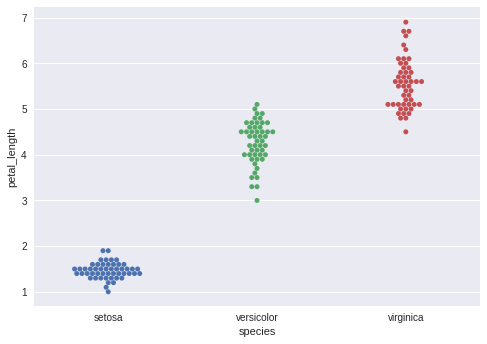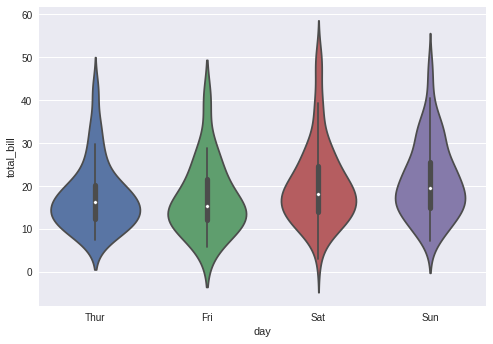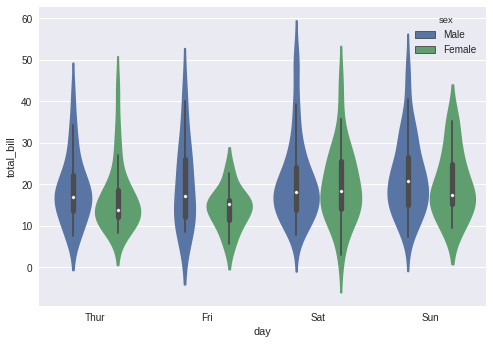# Seaborn - How to work with Distribution of Observations¶

In categorical scatter plots which we dealt with in the previous chapter, the approach becomes limited in the information it can provide about the distribution of values within each category. Now, going further, let us see what can facilitate us with performing comparisons within categories.

Box Plots Boxplot is a convenient way to visualize the distribution of data through their quartiles.

Box plots usually have vertical lines extending from the boxes which are termed as whiskers. These whiskers indicate variability outside the upper and lower quartiles, hence Box Plots are also termed as box-and-whisker plot and box-and-whisker diagram. Any Outliers in the data are plotted as individual points.

In :
import pandas as pd
import seaborn as sb
from matplotlib import pyplot as plt
sb.swarmplot(x = "species", y = "petal_length", data = df)
plt.show()The dots on the plot indicates the outlier.

### Violin Plots¶

Violin Plots(are a combination of the box plot with the kernel density estimates. So, these plots are easier to analyze and understand the distribution of the data.

Let us use the tips dataset called to learn more into violin plots. This dataset contains information related to the tips given by the customers in a restaurant.

In :
import pandas as pd
import seaborn as sb
from matplotlib import pyplot as plt
sb.violinplot(x = "day", y = "total_bill", data=df)
plt.show()The quartile and whisker values from the boxplot are shown inside the violin. As the violin plot uses KDE, the wider portion of the violin indicates the higher density and narrow region represent relatively lower density. The Inter-Quartile range in boxplot and higher density portion in KDE fall in the same region of each category of the violin plot.

The above plot shows the distribution of total_bill on four days of the week. But, in addition to that, if we want to see how the distribution behaves with respect to sex, let’s explore it in the below example.

In :
import pandas as pd
import seaborn as sb
from matplotlib import pyplot as plt
sb.violinplot(x = "day", y = "total_bill",hue = 'sex', data = df)
plt.show()Now we can clearly see the spending behavior between males and females. We can easily say that men make more bills than women by looking at the plot.

And, if the hue variable has only two classes, we can beautify the plot by splitting each violin into two instead of two violins on a given day. Either parts of the violin refer to each class in the hue variable.

In :
import pandas as pd
import seaborn as sb
from matplotlib import pyplot as pltSunny Solanki